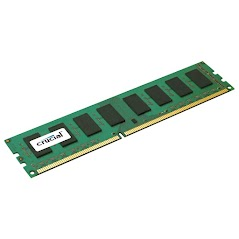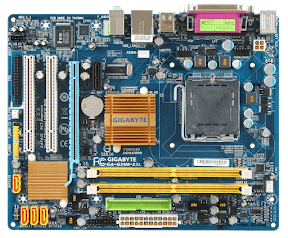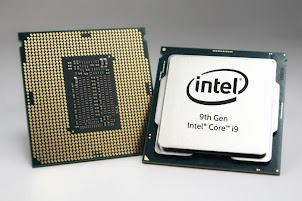Computer Hardware & Software Quiz
First & Last Name *
Which of the following is the collection of physical parts of a computer? *
1 point
Which of the following is an example of an operating system? *
1 point
Which of the following instructs computer hardware how to operate? *
1 point
Which of the following is used to protect a computer's internal hardware from physical damage? *
1 point
Which of the following connects to every component within a computer? *
1 point
A _________ acts like the brain of the computer quickly calculating a series of ones and zeros (binary data). *
1 point
Which of the following is a removable storage device that is measured in bytes? *
1 point
Which of the following stores information that the computer is not currently accessing? *
1 point
Which of the following stores information that the computer is currently accessing? *
1 point
Which of the following is measured in hertz (Hz)? *
1 point
Which of the following is an example of an output device? *
1 point
Which of the following is an example of an input device? *
1 point
Which of the following is the pictured below? *
1 pointWhich of the following is the pictured below? *
1 pointWhich of the following is the pictured below? *
1 point